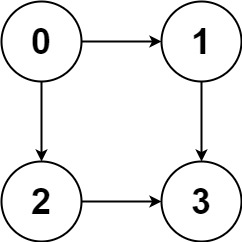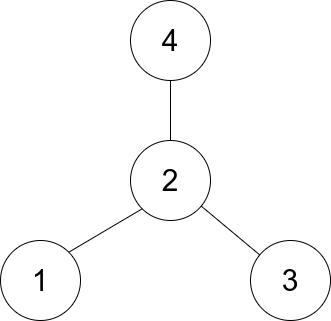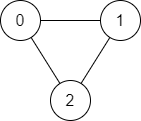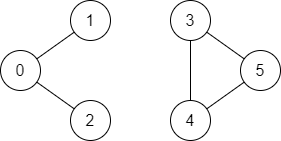## Pattern: Graphs

### [797/Medium] All Paths From Source to Target

#### Problem

• Given a directed acyclic graph (DAG) of n nodes labeled from 0 to n - 1, find all possible paths from node 0 to node n - 1 and return them in any order.

• The graph is given as follows: graph[i] is a list of all nodes you can visit from node i (i.e., there is a directed edge from node i to node graph[i][j]).

• Example 1:Input: graph = [[1,2],,,[]]
Output: [[0,1,3],[0,2,3]]
Explanation: There are two paths: 0 -> 1 -> 3 and 0 -> 2 -> 3.

• Example 2:Input: graph = [[4,3,1],[3,2,4],,,[]]
Output: [[0,4],[0,3,4],[0,1,3,4],[0,1,2,3,4],[0,1,4]]

• Constraints:
• n == graph.length
• 2 <= n <= 15
• 0 <= graph[i][j] < n
• graph[i][j] != i (i.e., there will be no self-loops).
• All the elements of graph[i] are unique.
• The input graph is guaranteed to be a DAG.
• See problem on LeetCode.

#### Solution: DFS

• If it asks for just the number of paths, we can generally solve it in two ways:
• Count from start to target in topological order.
• Count using DFS with memo.
• Note that both of them have time $$O(Edges)$$ and space $$O(Nodes)$$.
• This problem asks for all paths. Memo might not save much time.
• Imagine the worst case that we have $$node-1$$ to $$node-N$$, and $$node-i$$ linked to $$node-j$$ if $$i < j$$.
• There are $$2^(N-2)$$ paths and $$(N+2)*2^(N-3)$$ nodes in all paths. We can roughly say $$O(2^N)$$.
class Solution:
def allPathsSourceTarget(self, graph: List[List[int]]) -> List[List[int]]:
def dfs(cur, path):
if cur == len(graph) - 1:
res.append(path)
else:
for i in graph[cur]:
dfs(i, path + [i])

res = []
dfs(0, )
return res


#### Solution: Recursive One-liner

class Solution:
def allPathsSourceTarget(self, g, cur=0):
if cur == len(g) - 1:
return [[len(g) - 1]]

return [([cur] + path) for i in g[cur] for path in self.allPathsSourceTarget(g, i)]

##### Complexity
• Time: $$O(n)$$
• Space: $$O(1)$$

### [997/Easy] Find the Town Judge

#### Problem

• In a town, there are n people labeled from 1 to n. There is a rumor that one of these people is secretly the town judge.

• If the town judge exists, then:

• The town judge trusts nobody.
• Everybody (except for the town judge) trusts the town judge.
• There is exactly one person that satisfies properties 1 and 2.
• You are given an array trust where trust[i] = [a_i, b_i] representing that the person labeled a_i trusts the person labeled bi.

• Return the label of the town judge if the town judge exists and can be identified, or return -1 otherwise.

• Example 1:

Input: n = 2, trust = [[1,2]]
Output: 2

• Example 2:
Input: n = 3, trust = [[1,3],[2,3]]
Output: 3

• Example 3:
Input: n = 3, trust = [[1,3],[2,3],[3,1]]
Output: -1

• Constraints:
• 1 <= n <= 1000
• 0 <= trust.length <= 104
• trust[i].length == 2
• All the pairs of trust are unique.
• a_i != b_i
• 1 <= a_i, b_i <= n
• See problem on LeetCode.

#### Solution: Maintain a score for each person to be a town judge candidate; add/subtract one if the person is trusted/trusts; check for count

from collections import Counter

class Solution:
def findJudge(self, n: int, trust: List[List[int]]) -> int:
# base case: early termination
if n == 1 and trust == []:
return 1

# score for each person to be a town judge candidate
score = Counter()

# if a person trusts another person, decrease their score by one
# (since the town judge trusts nobody)
# if a person is trusted, increase their score by one
# (since everybody trusts the town judge)
for a, b in trust:
score[a] -= 1
score[b] += 1

# count number of people which trust the candidates
# if n-1 people trust one candidate it is the town judge
for i in range(1, n + 1):
if score[i] == n - 1:
return i

return -1

##### Complexity
• Time: $$O(n)$$
• Space: $$O(1)$$

### [1791/Easy] Find Center of Star Graph

#### Problem

• There is an undirected star graph consisting of n nodes labeled from 1 to n. A star graph is a graph where there is one center node and exactly n - 1 edges that connect the center node with every other node.

• You are given a 2D integer array edges where each edges[i] = [u_i, v_i] indicates that there is an edge between the nodes u_i and v_i. Return the center of the given star graph.

• Example 1:Input: edges = [[1,2],[2,3],[4,2]]
Output: 2
Explanation: As shown in the figure above, node 2 is connected to every other node, so 2 is the center.

• Example 2:
Input: edges = [[1,2],[5,1],[1,3],[1,4]]
Output: 1

• Constraints:
• 3 <= n <= 105
• edges.length == n - 1
• edges[i].length == 2
• 1 <= ui, vi <= n
• ui != vi
• The given edges represent a valid star graph.
• See problem on LeetCode.

#### Solution: Check the first two edges and return the overlapping node

• The solution is based on the following points:
• The center is the only node that has more than one edge.
• The center is also connected to all other nodes.
• Any two edges must have a common node, which is the center.
• We can only check the first two edges and return the common node:
class Solution:
def findCenter(self, edges: List[List[int]]) -> int:
for i in edges:
if i in edges:
return i

class Solution:
def findCenter(self, edges: List[List[int]]) -> int:
return edges if edges == edges or edges == edges else edges

##### Complexity
• Time: $$O(1)$$
• Space: $$O(1)$$

#### Solution: Generalized version: find multiple centers in a multi-star graph

class Solution(object):
def findCenter(self, edges):
"""
:type edges: List[List[int]]
:rtype: int
"""
n = max(max(my_list) for my_list in edges)
adj_list = [[] for _ in range(n)]

for edge in edges:

return i +1

##### Complexity
• Time: $$O(n)$$
• Space: $$O(1)$$

### [1971/Easy] Find if Path Exists in Graph

#### Problem

• There is a bi-directional graph with n vertices, where each vertex is labeled from 0 to n - 1 (inclusive). The edges in the graph are represented as a 2D integer array edges, where each edges[i] = [u_i, v_i] denotes a bi-directional edge between vertex u_i and vertex v_i. Every vertex pair is connected by at most one edge, and no vertex has an edge to itself.

• You want to determine if there is a valid path that exists from vertex source to vertex destination.

• Given edges and the integers n, source, and destination, return true if there is a valid path from source to destination, or false otherwise.

• Example 1:Input: n = 3, edges = [[0,1],[1,2],[2,0]], source = 0, destination = 2
Output: true
Explanation: There are two paths from vertex 0 to vertex 2:
- 0 → 1 → 2
- 0 → 2

• Example 2:Input: n = 6, edges = [[0,1],[0,2],[3,5],[5,4],[4,3]], source = 0, destination = 5
Output: false
Explanation: There is no path from vertex 0 to vertex 5.

• Constraints:
• 1 <= n <= 2 * 105
• 0 <= edges.length <= 2 * 105
• edges[i].length == 2
• 0 <= ui, vi <= n - 1
• ui != vi
• 0 <= source, destination <= n - 1
• There are no duplicate edges.
• There are no self edges.
• See problem on LeetCode.

#### Solution: DFS

class Solution:
def validPath(self, n: int, edges: List[List[int]], source: int, destination: int) -> bool:
graph = self.makeGraph(edges)
return self.depthFirstSearch(graph, source, destination, set())

# format: {x: [y], y: [x]}
def makeGraph(self,edges):
graph = {}

for edge in edges:
x,y = edge

if x not in graph:
graph[x] = []
if y not in graph:
graph[y] = []

graph[x].append(y)
graph[y].append(x)
return graph

def depthFirstSearch(self, graph, node, target, visited):
# base case: reached target
if node == target:
return True

# mark visited nodes

• Time: $$O(n)$$
• Space: $$O(2n + n + n) = O(n)$$